Function Repository Resource:

# SkewnessAround

Calculate the skewness of a list along with its uncertainty

Contributed by: Sander Huisman
 ResourceFunction["SkewnessAround"][{x1,x2,x3,…}] gives an Around object describing the skewness and corresponding uncertainty of the xi.

## Details

The standard error of the skewness is defined as: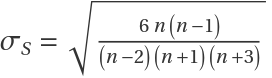, where n is the number of samples.
The "Value" of the Around object is calculated using the built-in Skewness function.

## Examples

### Basic Examples (1)

Calculate the skewness of some numbers:

 In:=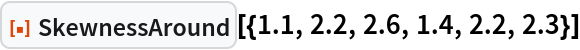Out=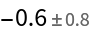### Scope (2)

SkewnessAround works with symbolic expressions:

 In:=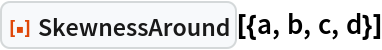Out=SkewnessAround also works with quantities with units, though the answer is dimensionless:

 In:=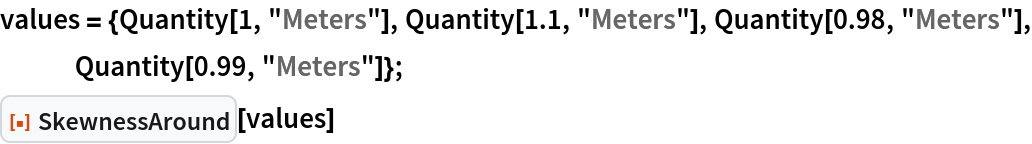Out=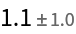The skewness is independent of the units of course:

 In:=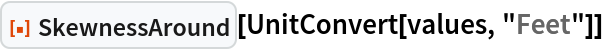Out=### Possible Issues (3)

SkewnessAround applied to symbolic expressions can get unwieldy fast:

 In:=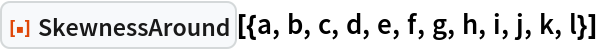Out=At least three value are needed to give a plausible error estimate:

 In:=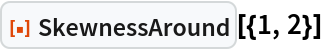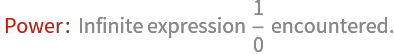Out=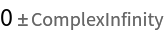Try with 3 values:

 In:=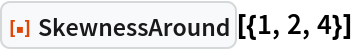Out=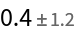Skewness can give Indeterminate, in which case there will not be an Around result: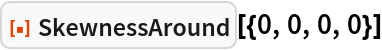### Neat Examples (1)

Study the skewness and its uncertainty for a sequence of values and compare it to the theoretical value of the distribution (dashed):

 In:=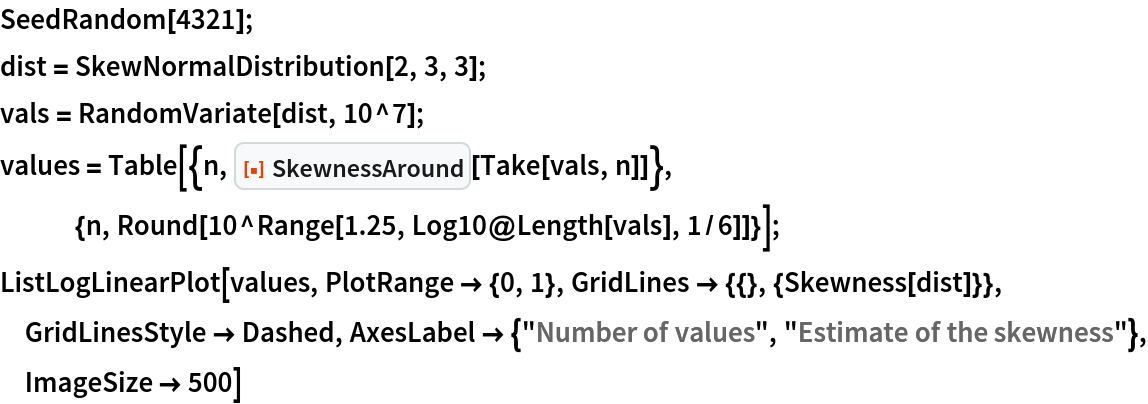Out=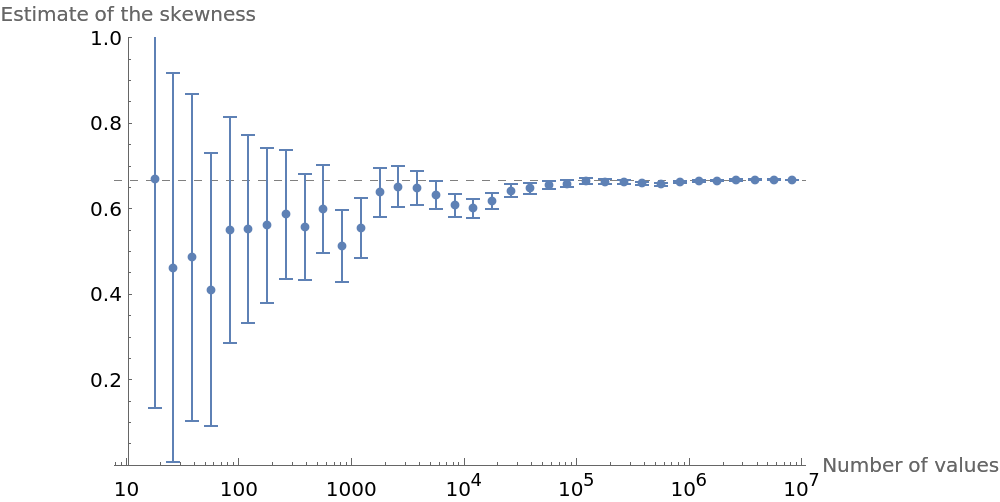SHuisman

## Version History

• 1.0.0 – 21 November 2022